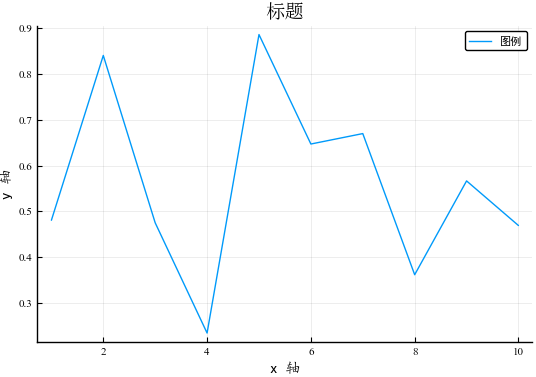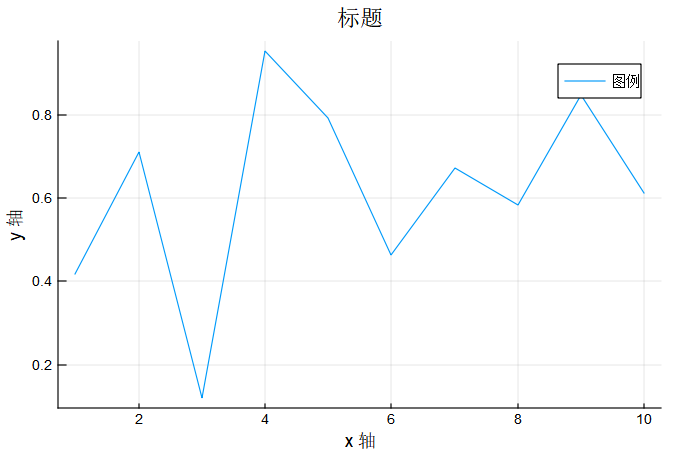# 利用Plots包可视化数据，如何显示中文标题、图例、坐标等？

Plots具有强大的可视化功能，同时也具有丰富的背景选项，但是似乎无法正常显示中文标题、中文图例和中文坐标等，感觉中文兼容性始终是个bug.

1. 尝试了一下，在 pyplot、plotly 和 gr 后端中，貌似只有 plotly 对中文支持不错 ↓
``````using Plots
``````
``````plotly()   # plotly backends
``````
``````plot(1:10, rand(10), title="标题", xlabel="x 轴", ylabel="y 轴",
label="图例", xtickfont = font(15, "Courier"))
``````

1. 但是 plotly 在使用数组 label 作图例的时候有问题 ↓

pyplot 后端 ↓

gr 后端支持数组图例 ↓

【Julia 0.6 环境，Plots: 0.16.0】

plotly()
plot(rand(10,2), label = [“aa” “bb”])

titlefont, tickfont, legendfont, guidefont, legendtitlefont.

1. 在pyplot里面用中文比较简单，只要在matplotlibrc里面加入默认中文字体就好了，或者将上述每个字体都设置好。
`font.sans-serif : Microsoft YaHei`

``````using Plots
pyplot()
plot(rand(10), title="标题", xlabel="x 轴", ylabel="y 轴",
label="图例",titlefont="SimSun",tickfont="Times New Roman",legendfont="SimHei",guidefont="KaiTi")
``````1. 对于gr，由于gr默认的编码是Latin-1，所以需要先给他调成utf-8。但是测试发现gr的字体好像不太好调。。反正像pyplot那样直接调是不行的，默认的只有宋体，有点丑了。。

``````ENV["GKS_ENCODING"] = "utf-8"
using Plots
plot(1:10, rand(10), title="标题", xlabel="x 轴", ylabel="y 轴", label="图例")
``````2赞